Скачать презентацию CS 100 A Lect 11 6 Oct 1998

0ba950918f8c80cfdaf3b7cfaeaa9731.ppt

• Количество слайдов: 12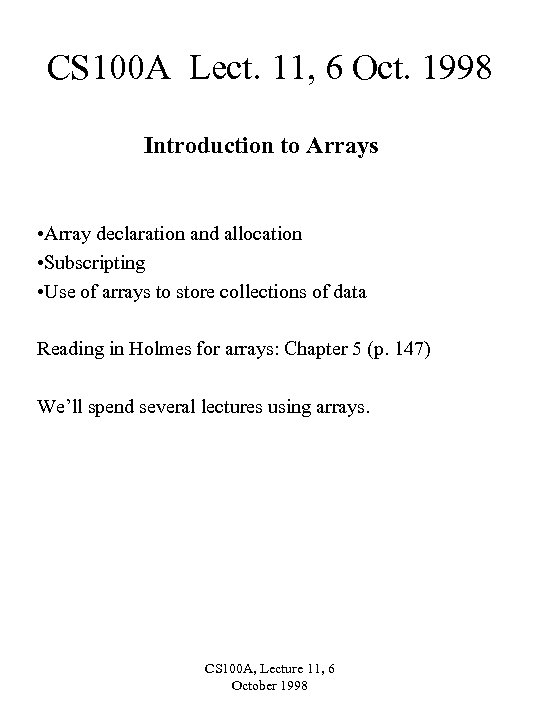CS 100 A Lect. 11, 6 Oct. 1998 Introduction to Arrays • Array declaration and allocation • Subscripting • Use of arrays to store collections of data Reading in Holmes for arrays: Chapter 5 (p. 147) We’ll spend several lectures using arrays. CS 100 A, Lecture 11, 6 October 1998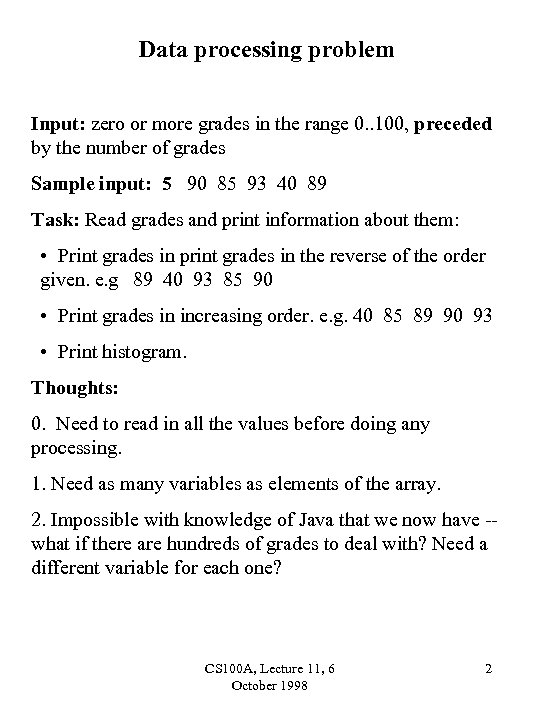Data processing problem Input: zero or more grades in the range 0. . 100, preceded by the number of grades Sample input: 5 90 85 93 40 89 Task: Read grades and print information about them: • Print grades in print grades in the reverse of the order given. e. g 89 40 93 85 90 • Print grades in increasing order. e. g. 40 85 89 90 93 • Print histogram. Thoughts: 0. Need to read in all the values before doing any processing. 1. Need as many variables as elements of the array. 2. Impossible with knowledge of Java that we now have -what if there are hundreds of grades to deal with? Need a different variable for each one? CS 100 A, Lecture 11, 6 October 1998 2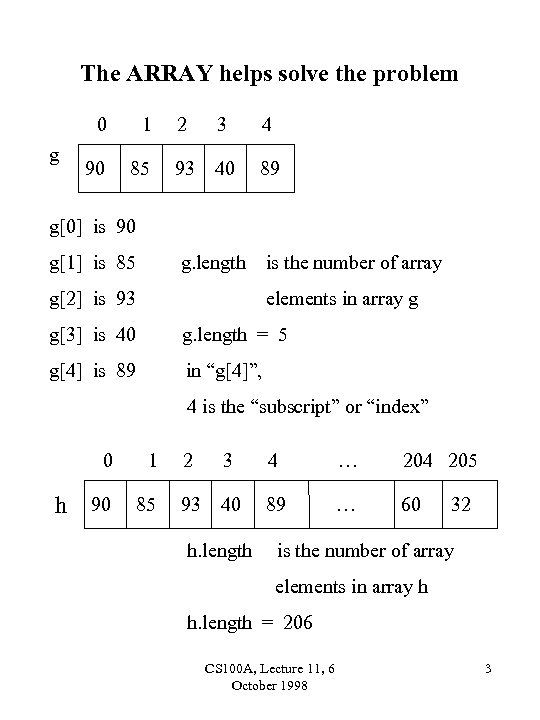The ARRAY helps solve the problem 0 g 1 90 85 2 3 4 93 40 89 g is 90 g is 85 g. length g is 93 is the number of array elements in array g g is 40 g. length = 5 g is 89 in “g”, 4 is the “subscript” or “index” 0 1 h 90 85 2 3 4 … 204 205 93 40 89 … 60 h. length 32 is the number of array elements in array h h. length = 206 CS 100 A, Lecture 11, 6 October 1998 3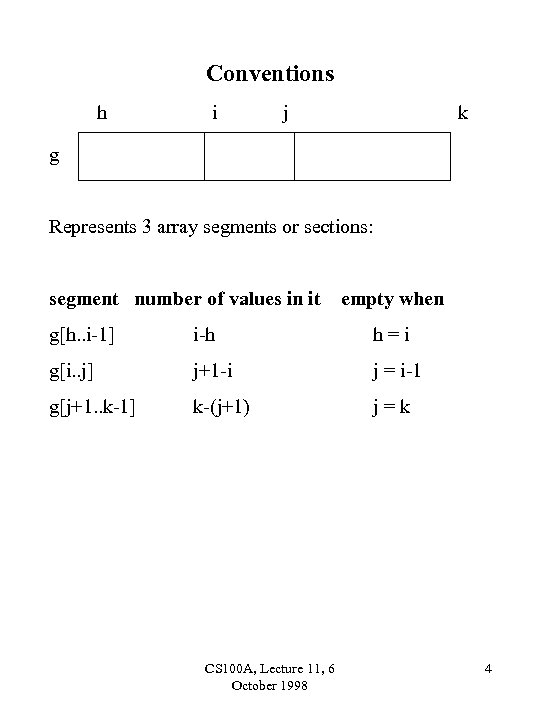Conventions h i j k g Represents 3 array segments or sections: segment number of values in it empty when g[h. . i-1] i-h h=i g[i. . j] j+1 -i j = i-1 g[j+1. . k-1] k-(j+1) j=k CS 100 A, Lecture 11, 6 October 1998 4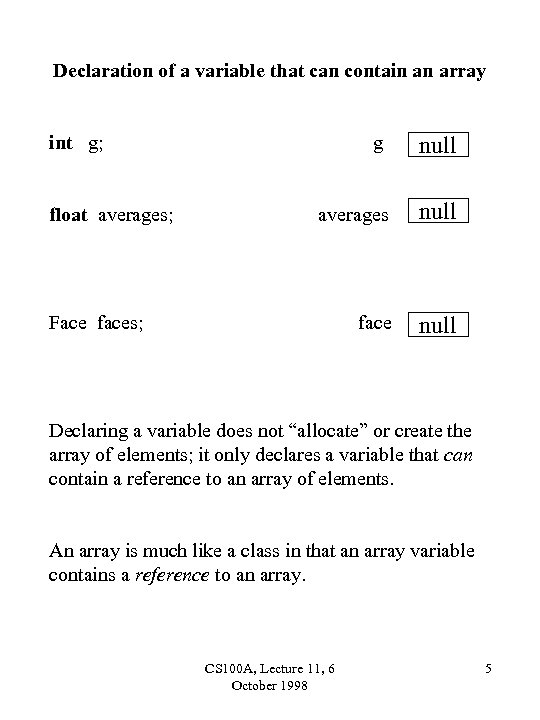Declaration of a variable that can contain an array int g; float averages; g null averages null face null Face faces; Declaring a variable does not “allocate” or create the array of elements; it only declares a variable that can contain a reference to an array of elements. An array is much like a class in that an array variable contains a reference to an array. CS 100 A, Lecture 11, 6 October 1998 5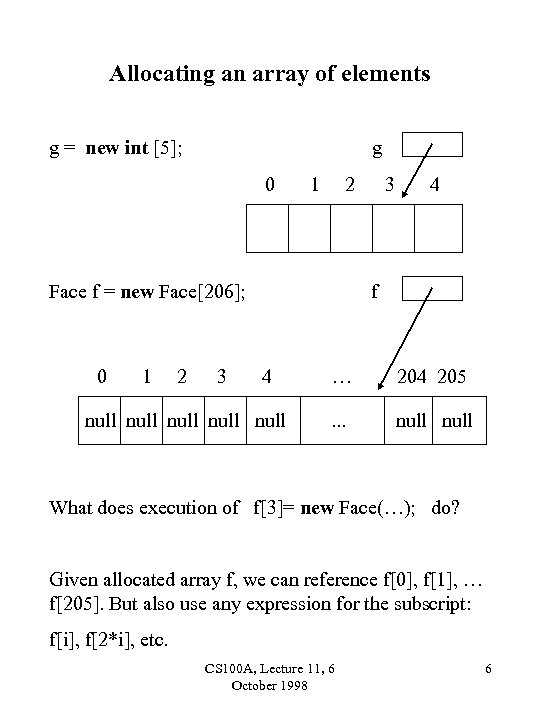Allocating an array of elements g = new int ; g 0 1 2 Face f = new Face; 0 1 2 3 3 4 f 4 null null … 204 205 . . . null What does execution of f= new Face(…); do? Given allocated array f, we can reference f, f, … f. But also use any expression for the subscript: f[i], f[2*i], etc. CS 100 A, Lecture 11, 6 October 1998 6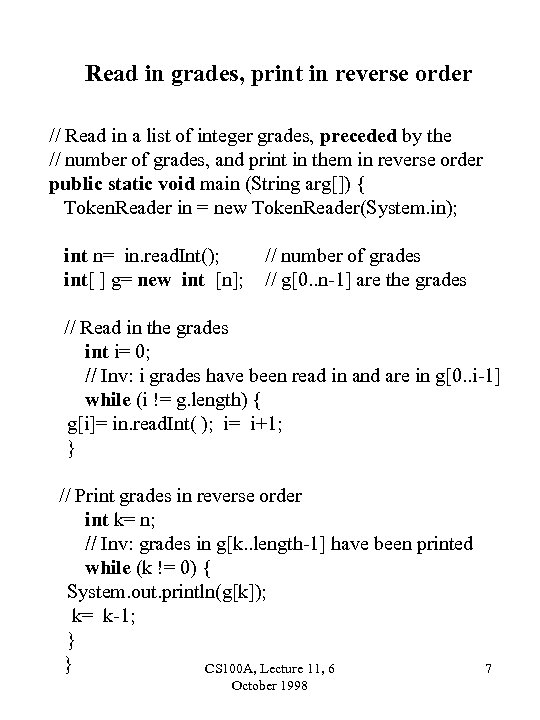Read in grades, print in reverse order // Read in a list of integer grades, preceded by the // number of grades, and print in them in reverse order public static void main (String arg[]) { Token. Reader in = new Token. Reader(System. in); int n= in. read. Int(); int[ ] g= new int [n]; // number of grades // g[0. . n-1] are the grades // Read in the grades int i= 0; // Inv: i grades have been read in and are in g[0. . i-1] while (i != g. length) { g[i]= in. read. Int( ); i= i+1; } // Print grades in reverse order int k= n; // Inv: grades in g[k. . length-1] have been printed while (k != 0) { System. out. println(g[k]); k= k-1; } } CS 100 A, Lecture 11, 6 October 1998 7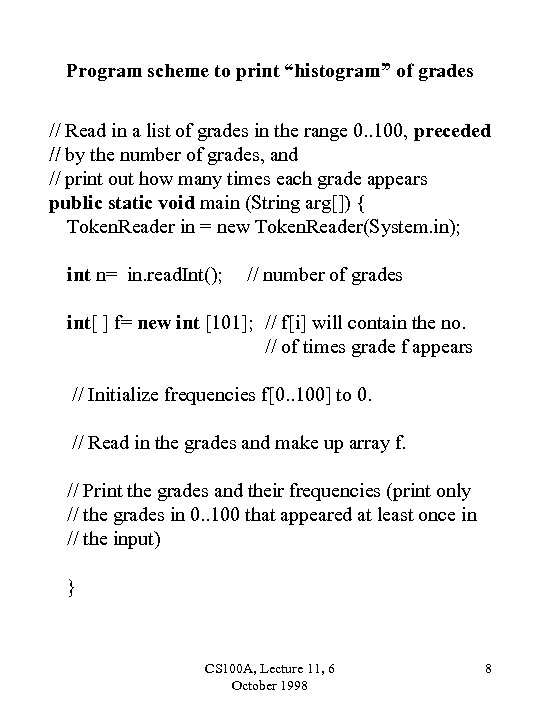Program scheme to print “histogram” of grades // Read in a list of grades in the range 0. . 100, preceded // by the number of grades, and // print out how many times each grade appears public static void main (String arg[]) { Token. Reader in = new Token. Reader(System. in); int n= in. read. Int(); // number of grades int[ ] f= new int ; // f[i] will contain the no. // of times grade f appears // Initialize frequencies f[0. . 100] to 0. // Read in the grades and make up array f. // Print the grades and their frequencies (print only // the grades in 0. . 100 that appeared at least once in // the input) } CS 100 A, Lecture 11, 6 October 1998 8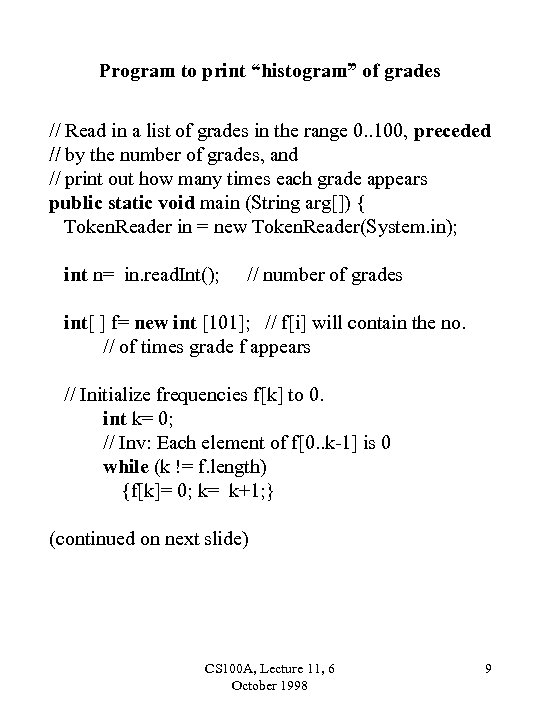Program to print “histogram” of grades // Read in a list of grades in the range 0. . 100, preceded // by the number of grades, and // print out how many times each grade appears public static void main (String arg[]) { Token. Reader in = new Token. Reader(System. in); int n= in. read. Int(); // number of grades int[ ] f= new int ; // f[i] will contain the no. // of times grade f appears // Initialize frequencies f[k] to 0. int k= 0; // Inv: Each element of f[0. . k-1] is 0 while (k != f. length) {f[k]= 0; k= k+1; } (continued on next slide) CS 100 A, Lecture 11, 6 October 1998 9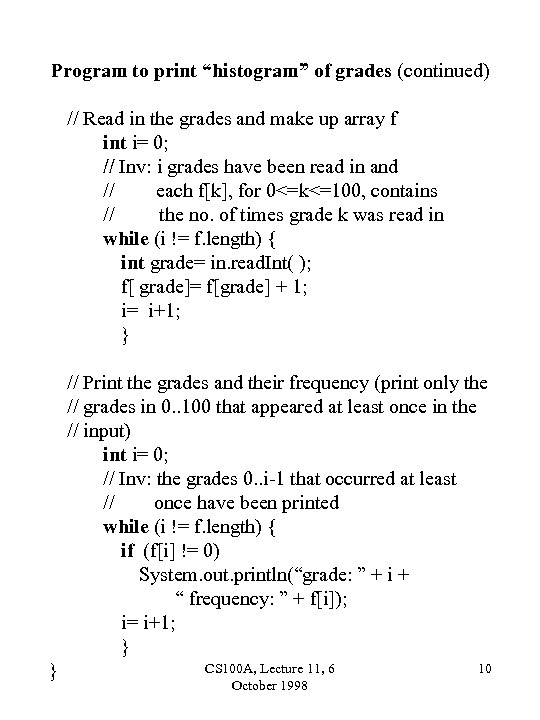Program to print “histogram” of grades (continued) // Read in the grades and make up array f int i= 0; // Inv: i grades have been read in and // each f[k], for 0<=k<=100, contains // the no. of times grade k was read in while (i != f. length) { int grade= in. read. Int( ); f[ grade]= f[grade] + 1; i= i+1; } // Print the grades and their frequency (print only the // grades in 0. . 100 that appeared at least once in the // input) int i= 0; // Inv: the grades 0. . i-1 that occurred at least // once have been printed while (i != f. length) { if (f[i] != 0) System. out. println(“grade: ” + i + “ frequency: ” + f[i]); i= i+1; } } CS 100 A, Lecture 11, 6 October 1998 10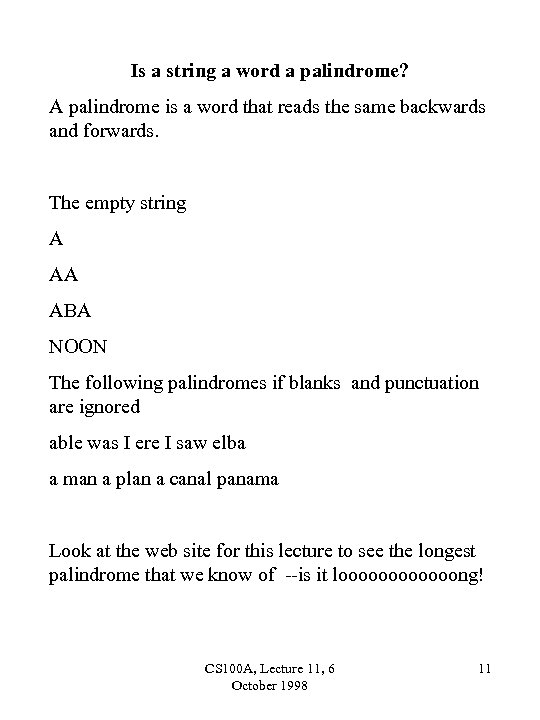Is a string a word a palindrome? A palindrome is a word that reads the same backwards and forwards. The empty string A AA ABA NOON The following palindromes if blanks and punctuation are ignored able was I ere I saw elba a man a plan a canal panama Look at the web site for this lecture to see the longest palindrome that we know of --is it loooooong! CS 100 A, Lecture 11, 6 October 1998 11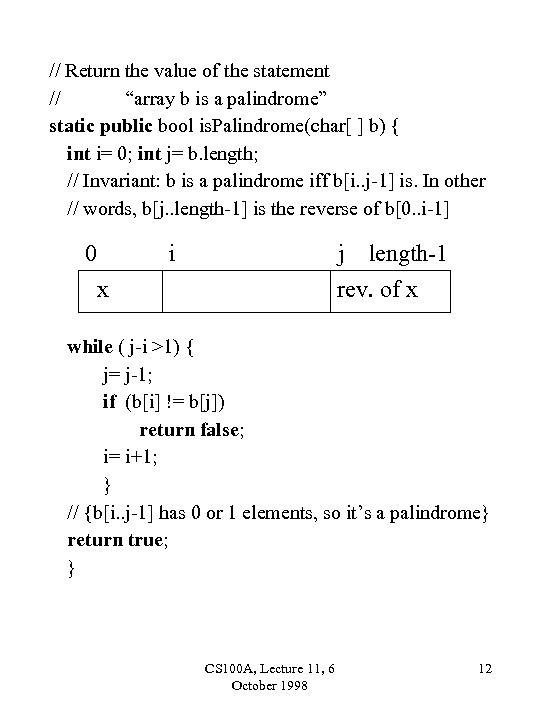// Return the value of the statement // “array b is a palindrome” static public bool is. Palindrome(char[ ] b) { int i= 0; int j= b. length; // Invariant: b is a palindrome iff b[i. . j-1] is. In other // words, b[j. . length-1] is the reverse of b[0. . i-1] 0 i j x length-1 rev. of x while ( j-i >1) { j= j-1; if (b[i] != b[j]) return false; i= i+1; } // {b[i. . j-1] has 0 or 1 elements, so it’s a palindrome} return true; } CS 100 A, Lecture 11, 6 October 1998 12